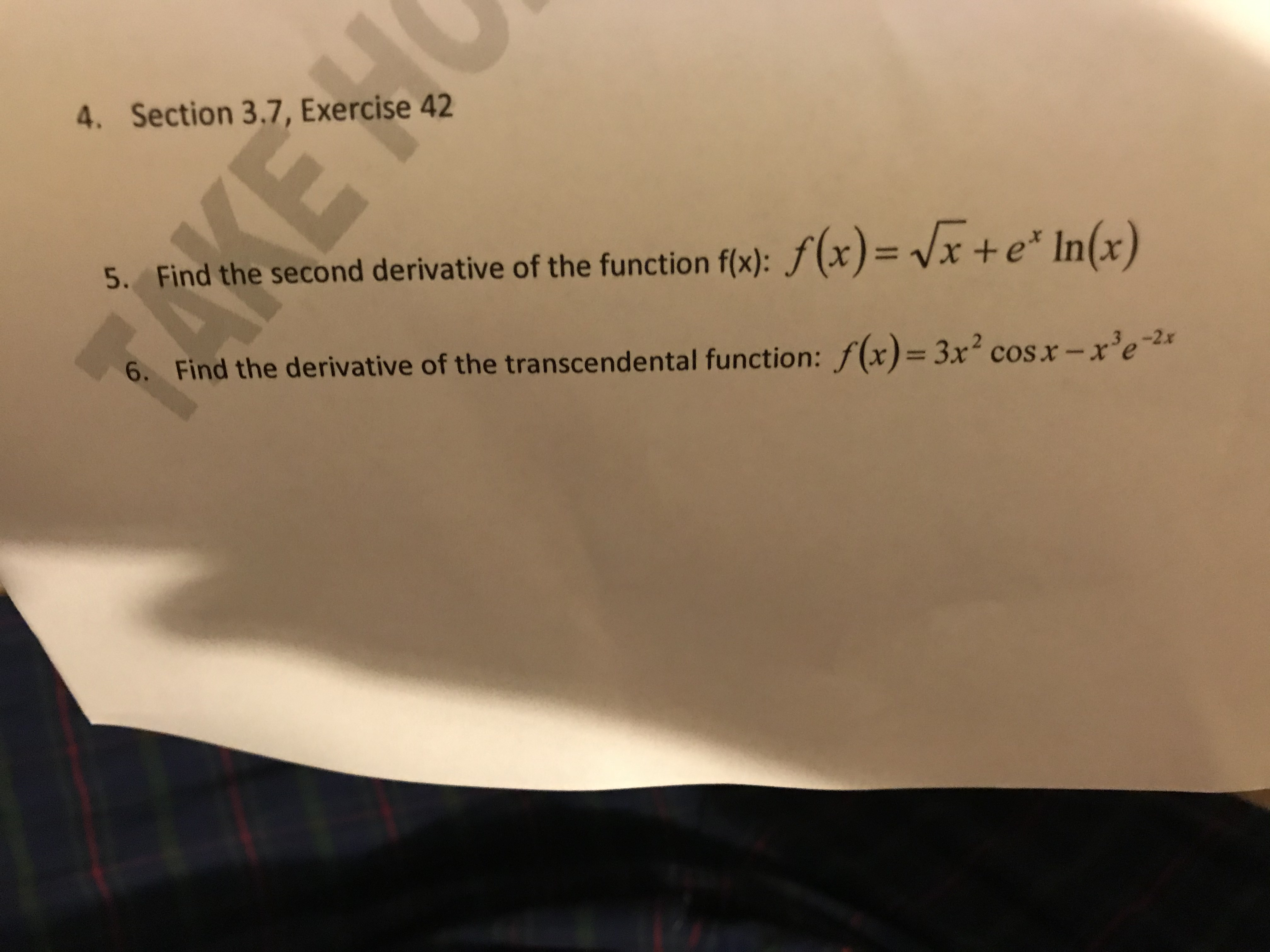# 4. Section 3.7, Exercise 42 5. Find the second derivative of the function f(x): f(x) = Vx + e* In(x) 6. Find the derivative of the transcendental function: f(x)= 3x2 cos x - x'e 2

Question

find derivative of the transcendental function

number 6help_outlineImage Transcriptionclose4. Section 3.7, Exercise 42 5. Find the second derivative of the function f(x): f(x) = Vx + e* In(x) 6. Find the derivative of the transcendental function: f(x)= 3x2 cos x - x'e 2 fullscreen

### Want to see this answer and more?

Experts are waiting 24/7 to provide step-by-step solutions in as fast as 30 minutes!*

*Response times vary by subject and question complexity. Median response time is 34 minutes and may be longer for new subjects.
Tagged in
MathCalculus

### Derivative